# Разработка ионно-плазменной технологической установки для нанесения функционального покрытия на крышки масляных фильтров, страница 37

We calculate pressure distribution on length of the pipeline. Effective pump speed is expressed by ratio:,                                              (4.17)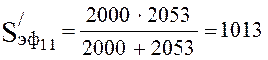l/s.

Pressure upon the input of the first section is defined by the equation:,                                                  (4.18)

where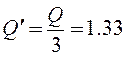l/s, as simultaneously works three pump lines.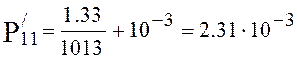torr.

Conductivity of the first section is described by expression: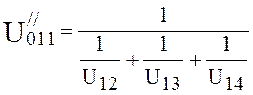,                                   (4.19)l/s.

Effective pump speed is defined by expression (4.17):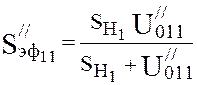.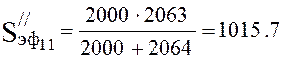l/s.

Pressure at the end of the first section is defined under the formula: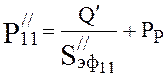,                                        (4.20)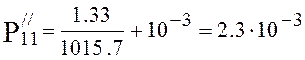torr.

Pressure at the beginning of the second section is equal to pressure upon the end of the first section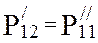. Conductivity of the pipeline is equal:,                                              (4.21)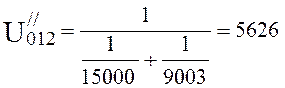l/s

Effective pump speed is defined by expression (4.17):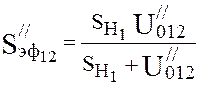.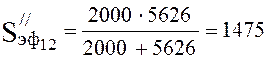l/s.

Pressure at the end of the second section is defined by expression (4.20):,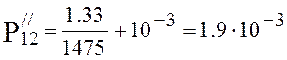torr.

Pressure at the beginning of the third section is equal to pressure upon the end of the second section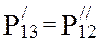. Conductivity of the pipeline is equal:,                                                         (4.22)l/s.

Effective pump speed is defined by expression (4.17):.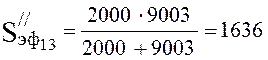l/s.

Pressure at the end of the third section is defined by expression (4.20):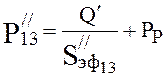,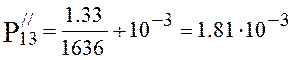torr.

Pressure at the beginning of the fourth section: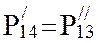. Conductivity of the pipeline is equal to infinity. Pressure at the end of the fourth section is defined by expression (4.20):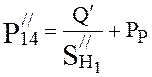,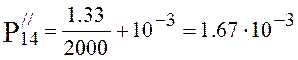torr.

The received results of pressure we bring in the table and build the schedule of pressure distribution on the pipeline length of the first section.

Table 4.3. Pressure distribution on the pipeline length of the first section of vacuum system

 N section L, m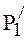, torr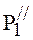, torr 1 object input -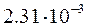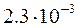2 pipeline 58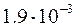3 valve 80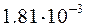4 pipeline 21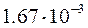5 TMP input -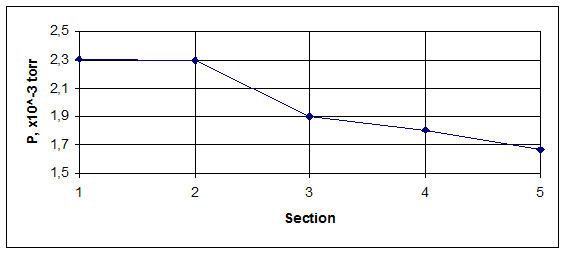Figure 4.2. Pressure distribution on the pipeline length of the first section of vacuum system.

### 4.2.2 Definition of design characteristics of the second  section of vacuum system

The second section of vacuum system represents consistently connected pipelines.

The scheme of a section is represented in figure 4.4.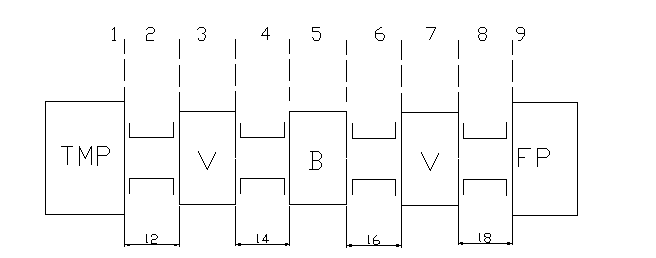Figure 4.3. The scheme of the second section of vacuum system

The general conductivity of the section of vacuum system from forepump DRYVAC 100S with conductivity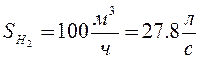up to TMP: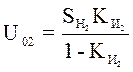,                                                        (4.23)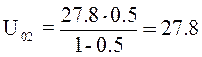l/s.

We define preliminary ratio between conductivities of separate sections: U21=U22=U24=U25=U26=U28=U, U23=U27=Uк=550 l/s. We determine the general conductivity of all section from expression:,           (4.24)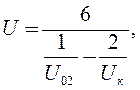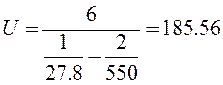l/s.

Constructively we appoint lengths of pipelines L2=30 sm, L4=8 sm, L6=55 sm, L8=37 sm.

We define a gas flow mode on the pipeline by working pressure of the vacuum pump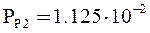torr and by diameter of an entrance branch pipe of the pump dвх=6.3 sm.

The free length of gas molecules (expressed in sm.) depending on pressure is defined by expression (4.10):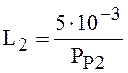,sm.

We define Knudsen criterion under the formula (4.11):,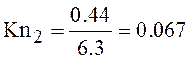.

The received result 1,5> Kn> 0.005, that corresponds to molecular-frictional mode of gas current. In this mode conductivity of the pipeline is defined under the formula: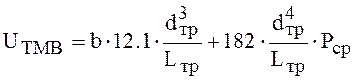, (4.25)